# Calculate totals in InfoPath

In this case I had to create an invoice request form. Users can request an invoice, that request will be send to the financial department where they process the invoice in the backend application.

I’ve set up a (form)document library in SharePoint to store the InfoPath files.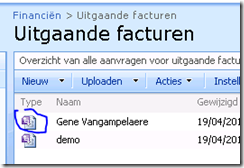Next step is to create the InfoPath form. You can use you own layout. In my example I had one recurring table to enter each article on the invoice.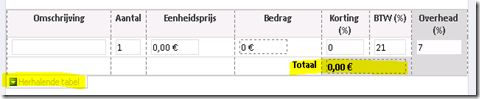As you can see I created 7 fields and a total field (in the table footer). The show the table footer right click on the table and select properties. In the view tab you enable the footer checkbox.

To calculate the totals I added one (hidden) field to the table and called it “subtotaal”.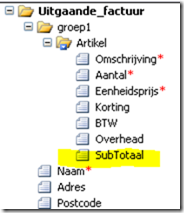That field will contain the formula to calculate the total for each article. In the footer we will use the sum formula to display the total.

Right click the subtotaal field and choose properties to enter the XPath formula: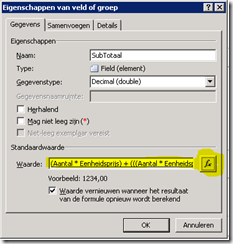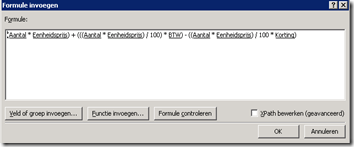In the footer of the table we add a calculated element and use the SUM formula: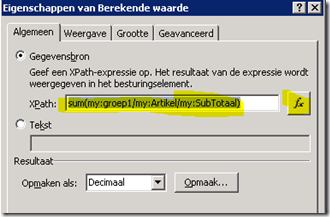This will do the trick.

A the end we publish the form to the created SharePoint library.